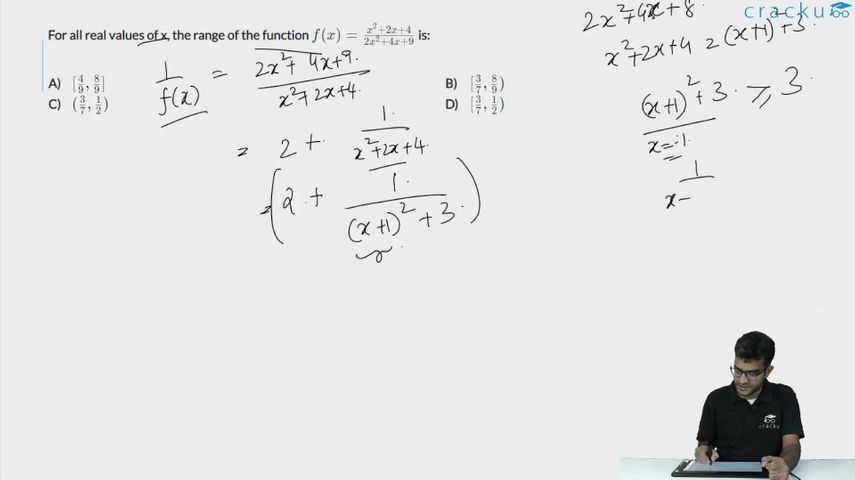Question 56

# For all real values of x, the range of the function $$f(x)=\frac{x^{2}+2x+4}{2x^{2}+4x+9}$$ is:

Solution

$$f(x)=\frac{x^{2}+2x+4}{2x^{2}+4x+9}$$

If we closely observe the coefficients of the terms in the numerator and denominator, we see that the coefficients of the $$x^2$$ and x in the numerators are in ratios 1:2. This gives us a hint that we might need to adjust the numerator to decrease the number of variables.

$$f(x)=\frac{x^2+2x+4}{2x^2+4x+9}=\frac{x^2+2x+4.5-0.5}{2x^2+4x+9}$$

= $$\frac{x^2+2x+4.5}{2x^2+4x+9}-\frac{0.5}{2x^2+4x+9}$$

= $$\frac{1}{2}-\frac{0.5}{2x^2+4x+9}$$

Now, we only have terms of x in the denominator.

The maximum value of the expression is achieved when the quadratic expression $$2x^2+4x+9$$ achieves its highest value, that is infinity.

In that case, the second term becomes zero and the expression becomes 1/2. However, at infinity, there is always an open bracket ')'.

To obtain the minimum value, we need to find the minimum possible value of the quadratic expression.

The minimum value is obtained when 4x + 4 = 0 [d/dx = 0]

x=-1.

The expression comes as 7.

The entire expression becomes 3/7.

Hence, $$[\frac{3}{7},\frac{1}{2})$$

### View Video Solution• All Quant CAT Formulas and shortcuts PDF
• 30+ CAT previous papers with solutions PDF